### The JokerHover over the thumbnail for a full-size version.

Author im_bad_at_N author:im_bad_at_n ibannart joker n-art rated supervillian 2009-08-08 2010-07-25 4 by 77 people. \$The Joker#im_bad_at_N#joker#00000000000000000000000000000000000000000000000000000000000000000000000000000000000000000000000000000000000000000000000000000000000000000000000000000000000000000000000000000000000000000000000000000000000000000000000000000000000000000000000000000000000000000000000000000000000000000000000000000000000000000000000000000000000000000000000000000000000000000000000000000000000000000000000000000000000000000000000000000000000000000000000000000000000000000000000000000000000000000000000000000000000000000000000000000000000000000000000000000000000000000000000000000000000000000000000000000000000000000000000000000000000000000000000000000000000000000000000000000000000000000000000000000000000000000000000000000000000000000|12^186,318!12^186,312!12^186,306!12^186,300!12^186,294!12^180,282!12^180,276!12^180,270!12^180,264!12^186,258!12^183,288!12^192,252!12^198,246!12^204,234!12^202,240!12^210,228!12^210,222!12^210,210!12^210,210!12^210,204!12^210,192!12^210,186!12^216,180!12^210,168!12^210,162!12^210,162!12^210,180!12^210,174!12^210,198!12^210,216!12^210,156!12^210,150!12^216,138!12^216,132!12^216,132!12^216,120!12^216,120!12^216,114!12^216,108!12^216,102!12^210,102!12^210,96!12^216,90!12^222,84!12^234,90!12^228,84!12^222,102!12^228,96!12^222,108!12^234,108!12^228,108!12^228,102!12^228,114!12^222,114!12^228,120!12^222,120!12^222,126!12^228,132!12^222,132!12^222,138!12^228,138!12^228,126!12^228,144!12^222,144!12^222,144!12^216,144!12^216,150!12^228,150!12^222,150!12^222,156!12^222,156!12^216,156!12^240,432!12^246,426!12^258,420!12^264,420!12^270,420!12^276,420!12^282,420!12^300,420!12^306,420!12^312,420!12^318,420!12^324,420!12^287,419!12^293,418!12^298,419!12^243,429!12^231,394!12^233,390!12^236,387!12^237,386!12^242,384!12^270,380!12^275,378!12^278,378!12^284,377!12^288,377!12^291,377!12^296,378!12^302,380!12^304,382!12^356,388!12^362,389!12^366,388!12^370,388!12^380,387!12^385,388!12^389,385!12^392,385!12^375,389!12^351,387!12^352,425!12^359,423!12^365,423!12^370,422!12^374,423!12^377,422!12^380,421!12^384,421!12^386,421!12^392,421!12^396,421!12^400,419!12^405,417!12^409,417!12^412,416!12^415,415!12^420,414!12^422,412!12^428,412!12^432,409!12^437,410!12^445,408!12^510,386!12^499,394!12^494,398!12^487,403!12^477,408!12^469,410!12^466,411!12^481,404!12^487,401!12^493,397!12^501,390!12^505,389!12^422,451!12^422,456!12^422,461!12^422,465!12^422,472!12^422,476!12^423,479!12^424,444!12^428,442!12^434,438!12^442,435!12^449,433!12^458,431!12^464,430!12^471,428!12^474,427!12^478,425!12^484,422!12^488,419!12^495,415!12^499,412!12^504,409!12^455,430!12^440,436!12^387,466!12^388,471!12^389,476!12^389,481!12^391,486!12^392,493!12^392,495!12^391,499!12^280,435!12^291,438!12^299,439!12^305,439!12^309,442!12^317,443!12^323,444!12^328,445!12^332,447!12^332,447!12^342,450!12^349,451!12^352,452!12^357,452!12^365,452!12^369,454!12^374,455!12^337,449!12^285,436!12^304,436!12^317,436!12^321,437!12^327,438!12^334,439!12^338,441!12^310,437!12^248,457!12^250,453!12^256,447!12^261,445!12^271,441!12^276,439!12^405,533!12^402,530!12^400,529!12^396,523!12^391,519!12^406,545!12^401,540!12^396,536!12^392,533!12^389,529!12^385,524!12^383,536!12^390,540!12^394,544!12^395,548!12^398,555!12^401,561!12^403,568!12^404,571!12^368,552!12^376,557!12^381,561!12^387,564!12^390,570!12^392,573!12^368,548!12^370,546!12^373,542!12^375,535!12^378,531!12^381,525!12^383,520!12^383,515!12^317,575!12^322,575!12^327,574!12^332,572!12^335,570!12^342,566!12^344,565!12^346,565!12^350,562!12^355,559!12^359,557!12^363,553!12^311,567!12^316,565!12^321,564!12^329,563!12^332,562!12^336,561!12^342,561!12^342,561!12^346,560!12^292,558!12^299,559!12^307,561!12^311,560!12^318,560!12^323,560!12^256,516!12^265,519!12^271,523!12^274,527!12^280,529!12^284,532!12^276,540!12^280,540!12^285,534!12^280,541!12^285,543!12^291,546!12^296,550!12^297,551!12^288,538!12^293,538!12^302,539!12^309,539!12^299,537!12^287,533!12^293,531!12^302,532!12^309,533!12^307,528!12^300,527!12^300,524!12^297,524!12^304,528!12^309,530!12^311,530!12^318,532!12^318,532!12^322,532!12^326,531!12^329,530!12^332,531!12^335,531!12^337,531!12^341,531!12^346,528!12^351,525!12^355,522!12^356,521!12^359,518!12^360,516!12^361,515!12^362,512!12^358,507!12^365,505!12^369,508!12^374,510!12^379,511!12^306,552!12^306,546!12^300,546!12^294,546!12^300,558!12^300,552!12^306,558!12^312,552!12^312,540!12^312,546!12^318,540!12^318,546!12^318,552!12^288,534!12^300,534!12^318,534!12^330,540!12^324,540!12^312,534!12^324,546!12^330,546!12^324,552!12^324,552!12^330,558!12^330,552!12^342,552!12^336,552!12^336,558!12^336,546!12^342,546!12^336,540!12^342,540!12^354,534!12^348,540!12^348,534!12^342,534!12^336,534!12^330,534!12^348,546!12^348,552!12^354,552!12^354,546!12^354,540!12^360,540!12^360,546!12^360,540!12^366,534!12^366,540!12^360,534!12^360,528!12^366,522!12^354,528!12^366,522!12^366,516!12^372,516!12^378,522!12^372,522!12^372,528!12^366,528!12^372,534!12^299,517!12^303,514!12^304,512!12^307,510!12^309,509!12^315,507!12^319,506!12^324,507!12^327,507!12^331,505!12^334,504!12^336,504!12^342,504!12^345,503!12^348,503!12^356,503!12^357,503!12^362,504!12^316,512!12^318,515!12^320,517!12^324,518!12^327,517!12^329,516!12^333,515!12^335,513!12^335,513!12^337,510!12^271,518!12^269,513!12^264,509!12^261,505!12^265,504!12^272,505!12^278,506!12^259,491!12^269,494!12^275,499!12^281,502!12^261,488!12^261,479!12^266,474!12^271,469!12^277,466!12^284,466!12^293,469!12^301,473!12^309,478!12^314,482!12^315,484!12^296,484!12^301,489!12^288,478!12^279,471!12^275,473!12^271,479!12^275,476!12^283,475!12^283,471!12^273,477!12^266,482!12^270,488!12^279,490!12^281,487!12^277,485!12^277,481!12^278,491!12^284,496!12^289,497!12^294,495!12^296,495!12^299,496!12^291,501!12^288,509!12^283,512!12^276,512!12^275,514!12^281,520!12^287,525!12^289,527!12^289,519!12^279,515!12^285,509!12^290,513!12^291,519!12^292,515!12^295,509!12^296,509!12^306,502!12^296,507!12^295,508!12^301,505!12^316,503!12^322,502!12^328,502!12^309,500!12^295,497!12^307,494!12^316,496!12^325,499!12^313,494!12^320,494!12^328,497!12^335,498!12^339,498!12^342,497!12^347,497!12^439,515!12^441,512!12^443,509!12^444,505!12^444,504!12^446,499!12^432,497!12^440,497!12^449,495!12^441,494!12^443,537!12^446,532!12^449,524!12^452,517!12^448,543!12^448,540!12^447,533!12^441,541!12^460,552!12^460,548!12^460,542!12^461,535!12^464,530!12^466,527!12^465,524!12^464,520!12^449,486!12^450,485!12^455,482!12^462,476!12^466,472!12^468,471!12^472,468!12^477,467!12^481,467!12^487,464!12^494,461!12^497,461!12^499,459!12^505,456!12^510,452!12^518,448!12^529,444!12^535,441!12^539,438!12^545,435!12^549,431!12^523,446!12^547,436!12^546,443!12^545,445!12^547,448!12^551,450!12^546,457!12^546,461!12^542,469!12^536,473!12^534,474!12^532,479!12^539,479!12^546,474!12^552,470!12^551,476!12^544,482!12^535,484!12^533,485!12^540,481!12^547,476!12^541,460!12^541,466!12^533,494!12^532,492!12^525,509!12^523,512!12^526,511!12^537,506!12^534,506!12^532,499!12^532,493!12^528,506!12^516,514!12^519,517!12^531,519!12^537,523!12^527,521!12^519,517!12^491,524!12^499,526!12^500,527!12^495,524!12^467,520!12^477,521!12^483,523!12^490,523!12^493,521!12^507,518!12^512,516!12^499,519!12^471,520!12^458,483!12^460,480!12^462,476!12^472,476!12^474,477!12^477,478!12^480,479!12^483,479!12^489,479!12^489,479!12^491,476!12^493,472!12^495,467!12^486,478!12^485,476!12^488,471!12^488,468!12^461,489!12^462,489!12^466,490!12^470,490!12^474,491!12^479,490!12^484,491!12^490,491!12^493,490!12^496,488!12^500,487!12^503,486!12^509,483!12^510,481!12^513,478!12^514,473!12^514,471!12^514,465!12^504,465!12^509,464!12^512,464!12^510,456!12^522,456!12^516,456!12^516,462!12^522,456!12^522,462!12^528,462!12^528,456!12^528,450!12^540,450!12^540,444!12^534,456!12^534,462!12^534,450!12^522,468!12^528,468!12^534,468!12^516,468!12^516,474!12^522,480!12^516,486!12^516,498!12^516,480!12^522,474!12^528,474!12^522,486!12^528,492!12^528,486!12^522,474!12^522,504!12^522,498!12^522,492!12^528,498!12^516,492!12^516,498!12^516,504!12^540,456!12^486,516!12^492,516!12^498,516!12^504,516!12^492,510!12^504,510!12^510,510!12^516,510!12^498,510!12^492,504!12^498,504!12^504,504!12^510,504!12^492,498!12^504,498!12^510,498!12^498,498!12^498,492!12^504,492!12^510,492!12^510,486!12^474,498!12^474,504!12^474,510!12^474,516!12^480,516!12^480,510!12^480,504!12^480,498!12^486,498!12^486,504!12^486,510!12^468,516!12^456,516!12^462,516!12^456,510!12^456,504!12^456,498!12^456,492!12^456,486!12^462,498!12^462,510!12^462,504!12^468,498!12^468,504!12^468,510!12^450,504!12^582,456!12^582,462!12^582,468!12^582,474!12^582,480!12^582,486!12^588,492!12^588,498!12^588,504!12^588,510!12^588,522!12^588,528!12^588,534!12^582,546!12^576,558!12^570,573!12^573,571!12^574,567!12^575,562!12^578,555!12^579,550!12^582,546!12^583,541!12^584,539!12^587,518!12^584,489!12^585,485!12^559,572!12^560,571!12^562,567!12^562,562!12^565,557!12^567,553!12^568,549!12^571,542!12^572,541!12^574,533!12^574,530!12^572,525!12^572,520!12^575,512!12^576,507!12^577,504!12^575,516!12^570,550!12^572,539!12^560,562!12^557,554!12^582,576!12^576,576!12^594,576!12^588,576!12^582,570!12^594,570!12^588,570!12^582,564!12^594,564!12^588,564!12^588,558!12^582,558!12^594,558!12^588,552!12^594,552!12^618,552!12^612,552!12^600,552!12^606,552!12^606,558!12^600,558!12^612,558!12^618,558!12^618,564!12^612,564!12^606,564!12^600,570!12^600,564!12^606,570!12^600,570!12^600,576!12^612,570!12^612,576!12^606,576!12^618,570!12^618,570!12^618,576!12^660,576!12^660,570!12^654,564!12^654,558!12^648,552!12^642,546!12^642,534!12^642,528!12^642,540!12^642,552!12^636,558!12^636,564!12^642,570!12^642,570!12^636,576!12^642,564!12^642,558!12^648,558!12^648,564!12^648,570!12^648,576!12^642,576!12^654,576!12^654,570!12^630,570!12^636,570!12^630,576!12^630,576!12^624,576!12^624,570!12^624,564!12^624,564!12^624,558!12^624,552!12^630,552!12^630,558!12^630,564!12^636,552!12^636,546!12^636,546!12^636,540!12^636,534!12^636,528!12^588,546!12^594,546!12^594,546!12^600,546!12^612,546!12^612,546!12^618,546!12^624,546!12^630,546!12^606,546!12^588,540!12^600,540!12^612,540!12^612,540!12^624,540!12^630,540!12^630,540!12^618,540!12^606,540!12^594,540!12^594,534!12^600,534!12^612,534!12^618,534!12^624,534!12^630,534!12^606,534!12^594,528!12^606,528!12^612,528!12^624,528!12^630,528!12^618,528!12^600,528!12^624,486!12^624,492!12^624,498!12^624,510!12^624,516!12^624,504!12^624,522!12^630,504!12^630,510!12^630,516!12^630,516!12^630,522!12^636,516!12^636,516!12^636,516!12^636,522!12^600,504!12^594,516!12^600,516!12^600,522!12^606,522!12^594,522!12^606,516!12^618,516!12^612,522!12^612,522!12^618,516!12^618,522!12^618,516!12^612,516!12^594,510!12^600,510!12^606,510!12^612,510!12^618,510!12^612,504!12^606,504!12^618,504!12^594,504!12^594,498!12^600,498!12^606,498!12^612,498!12^618,498!12^618,492!12^612,492!12^606,492!12^600,492!12^594,492!12^594,486!12^600,486!12^606,486!12^606,486!12^612,486!12^624,486!12^612,486!12^618,486!12^588,486!12^588,480!12^588,474!12^588,474!12^588,468!12^588,456!12^594,468!12^594,474!12^594,480!12^606,480!12^600,474!12^606,474!12^612,480!12^618,474!12^600,480!12^618,480!12^629,492!12^633,497!12^635,502!12^637,506!12^639,510!12^641,514!12^643,520!12^644,524!12^645,527!12^645,531!12^648,537!12^650,542!12^653,550!12^655,556!12^658,564!12^661,570!12^662,574!12^647,480!12^649,486!12^651,491!12^651,495!12^654,502!12^657,509!12^658,515!12^658,520!12^661,527!12^663,533!12^665,541!12^667,550!12^671,559!12^673,565!12^675,570!12^675,573!12^668,556!12^637,432!12^648,434!12^654,437!12^657,439!12^666,441!12^672,444!12^675,446!12^675,446!12^684,450!12^688,452!12^693,454!12^694,454!12^701,458!12^707,461!12^713,464!12^715,464!12^720,467!12^730,470!12^737,474!12^743,477!12^748,481!12^753,484!12^757,486!12^764,489!12^766,491!12^725,467!12^682,451!12^620,479!12^617,473!12^641,476!12^646,480!12^649,490!12^639,478!12^643,484!12^647,490!12^582,450!12^582,438!12^582,444!12^582,432!12^582,426!12^582,420!12^582,414!12^579,426!12^580,431!12^580,434!12^580,438!12^580,442!12^580,445!12^654,427!12^645,428!12^642,428!12^637,428!12^623,422!12^628,421!12^633,426!12^630,432!12^630,441!12^632,449!12^613,459!12^626,464!12^635,465!12^643,462!12^649,459!12^615,461!12^629,465!12^644,462!12^640,463!12^619,462!12^633,398!12^646,406!12^651,410!12^657,416!12^664,421!12^674,425!12^678,426!12^641,403!12^669,424!12^639,409!12^636,417!12^635,423!12^634,407!12^624,429!12^612,436!12^612,448!12^622,432!12^617,442!12^620,456!12^613,440!12^617,429!12^622,433!12^484,309!12^487,314!12^491,316!12^496,318!12^502,320!12^508,320!12^512,319!12^516,318!12^520,318!12^529,319!12^535,322!12^537,328!12^539,331!12^541,337!12^541,343!12^541,349!12^540,354!12^539,358!12^538,365!12^536,369!12^534,371!12^536,377!12^541,381!12^547,386!12^552,390!12^559,393!12^566,395!12^573,397!12^575,397!12^584,401!12^589,403!12^539,386!12^537,383!12^547,388!12^541,374!12^542,362!12^542,354!12^545,348!12^545,342!12^545,329!12^544,322!12^545,334!12^547,340!12^554,347!12^559,352!12^568,359!12^581,366!12^588,371!12^575,365!12^558,360!12^550,354!12^573,363!12^578,367!12^484,370!12^491,366!12^494,362!12^504,357!12^509,352!12^513,348!12^517,353!12^504,357!12^496,363!12^509,359!12^514,355!12^518,352!12^523,351!12^500,360!12^434,356!12^462,350!12^456,354!12^466,351!12^476,347!12^217,230!12^218,223!12^221,218!12^224,212!12^230,203!12^234,196!12^239,189!12^242,184!12^246,180!12^251,174!12^257,167!12^263,162!12^271,157!12^278,153!12^288,151!12^297,150!12^311,148!12^323,148!12^334,149!12^344,151!12^356,155!12^368,157!12^382,156!12^395,152!12^414,149!12^427,144!12^435,137!12^443,129!12^444,126!12^442,127!12^427,137!12^419,143!12^410,148!12^398,152!12^380,154!12^370,154!12^338,148!12^310,146!12^295,146!12^314,146!12^329,145!12^347,151!12^361,153!12^384,153!12^397,147!12^215,216!12^214,217!12^218,205!12^220,198!12^228,187!12^229,184!12^218,208!12^221,200!12^230,185!12^241,173!12^225,197!12^223,198!12^228,188!12^236,177!12^243,170!12^252,160!12^257,157!12^248,166!12^243,171!12^258,158!12^276,150!12^265,160!12^239,169!12^263,156!12^279,150!12^263,152!12^265,148!12^280,146!12^286,142!12^257,153!12^270,148!12^283,145!12^297,142!12^302,141!12^309,141!12^319,140!12^324,140!12^327,141!12^332,141!12^337,142!12^338,142!12^351,146!12^356,147!12^361,149!12^365,149!12^373,147!12^383,148!12^385,147!12^395,147!12^401,143!12^407,140!12^412,138!12^416,136!12^423,134!12^432,130!12^386,154!12^404,149!12^394,153!12^389,154!12^371,157!12^348,152!12^326,143!12^346,145!12^298,146!12^304,146!12^318,148!12^322,148!12^290,143!12^299,142!12^229,190!12^219,197!12^215,191!12^219,185!12^223,178!12^235,169!12^240,166!12^223,190!12^244,171!12^225,179!12^238,167!12^263,150!12^297,141!12^270,154!12^228,166!12^224,173!12^237,161!12^256,147!12^282,139!12^271,144!12^252,148!12^242,158!12^236,166!12^232,173!12^246,158!12^252,150!12^248,149!12^268,140!12^278,135!12^293,133!12^268,142!12^253,143!12^282,134!12^297,133!12^306,133!12^317,133!12^290,135!12^273,140!12^260,144!12^316,133!12^343,133!12^312,131!12^329,134!12^333,137!12^336,137!12^319,131!12^332,132!12^341,135!12^346,136!12^353,140!12^357,139!12^361,139!12^366,139!12^371,140!12^380,139!12^389,136!12^403,132!12^414,129!12^412,108!12^400,118!12^381,126!12^350,132!12^346,132!12^354,130!12^371,127!12^398,121!12^408,114!12^391,129!12^363,131!12^362,131!12^380,131!12^395,126!12^403,122!12^413,118!12^405,126!12^388,134!12^363,137!12^365,134!12^382,129!12^406,121!12^413,114!12^385,122!12^405,115!12^408,111!12^389,125!12^363,131!12^381,134!12^398,133!12^422,123!12^426,117!12^401,134!12^382,137!12^366,143!12^405,134!12^429,122!12^432,120!12^219,167!12^224,158!12^239,150!12^241,149!12^226,164!12^220,165!12^225,161!12^228,158!12^238,150!12^247,141!12^281,129!12^254,139!12^253,140!12^267,132!12^254,132!12^260,132!12^278,124!12^308,118!12^240,89!12^246,84!12^251,77!12^256,69!12^263,62!12^270,58!12^282,54!12^299,47!12^310,46!12^276,52!12^273,54!12^304,49!12^235,103!12^238,97!12^247,89!12^267,79!12^270,76!12^247,92!12^263,73!12^268,69!12^280,65!12^262,78!12^260,81!12^258,85!12^351,53!12^328,53!12^322,51!12^341,48!12^344,51!12^332,49!12^346,51!12^322,48!12^310,48!12^300,54!12^287,59!12^283,64!12^280,70!12^275,75!12^273,67!12^288,55!12^318,46!12^328,46!12^314,51!12^300,54!12^294,56!12^236,111!12^241,104!12^248,96!12^255,91!12^259,88!12^237,119!12^244,113!12^250,107!12^257,97!12^263,92!12^238,126!12^237,126!12^252,110!12^259,101!12^225,133!12^231,131!12^246,115!12^258,100!12^224,125!12^250,110!12^234,141!12^238,145!12^253,122!12^233,145!12^244,131!12^251,127!12^231,139!12^238,131!12^250,120!12^237,111!12^253,105!12^283,98!12^318,87!12^255,116!12^264,106!12^300,90!12^309,84!12^320,81!12^317,82!12^280,95!12^258,108!12^255,110!12^265,105!12^287,91!12^296,85!12^307,81!12^333,81!12^315,81!12^275,91!12^261,105!12^256,109!12^277,90!12^322,78!12^271,103!12^279,98!12^302,85!12^320,80!12^328,78!12^266,82!12^270,81!12^292,67!12^306,61!12^323,58!12^340,57!12^334,57!12^316,57!12^306,60!12^297,65!12^287,74!12^270,86!12^267,90!12^267,92!12^283,81!12^295,73!12^311,66!12^330,59!12^343,58!12^331,62!12^315,65!12^297,71!12^279,82!12^279,82!12^297,74!12^310,72!12^322,68!12^324,68!12^332,69!12^299,72!12^300,68!12^303,68!12^306,72!12^302,63!12^323,53!12^287,80!12^285,82!12^306,77!12^318,72!12^325,71!12^333,72!12^337,72!12^348,70!12^344,61!12^339,67!12^350,71!12^259,125!12^272,110!12^292,102!12^330,100!12^365,97!12^314,100!12^300,108!12^286,115!12^266,125!12^279,111!12^334,91!12^355,89!12^301,100!12^274,111!12^270,117!12^274,112!12^305,98!12^320,93!12^333,89!12^333,89!12^288,106!12^278,118!12^275,123!12^294,105!12^340,87!12^342,86!12^306,95!12^283,99!12^293,97!12^307,90!12^335,85!12^340,83!12^315,103!12^285,115!12^279,121!12^291,121!12^317,123!12^385,106!12^348,118!12^326,119!12^300,117!12^329,123!12^363,119!12^379,111!12^397,103!12^383,116!12^363,116!12^316,117!12^299,118!12^330,122!12^345,123!12^364,117!12^386,111!12^396,106!12^383,117!12^356,123!12^330,125!12^320,124!12^355,125!12^382,120!12^408,115!12^410,110!12^374,117!12^362,118!12^395,109!12^405,105!12^391,118!12^300,126!12^317,127!12^330,129!12^343,126!12^284,124!12^320,120!12^342,116!12^354,113!12^374,109!12^389,103!12^379,106!12^348,109!12^334,112!12^314,110!12^355,109!12^395,105!12^368,103!12^340,107!12^322,110!12^313,108!12^340,98!12^359,97!12^376,97!12^382,98!12^364,101!12^348,102!12^329,98!12^358,103!12^354,103!12^316,107!12^343,116!12^370,116!12^364,113!12^305,104!12^305,103!12^347,109!12^369,110!12^274,164!12^265,171!12^262,174!12^258,178!12^253,183!12^251,186!12^247,190!12^243,196!12^241,202!12^238,207!12^233,217!12^230,223!12^228,226!12^226,232!12^232,237!12^233,235!12^236,231!12^237,227!12^240,220!12^243,213!12^249,203!12^255,195!12^258,188!12^265,181!12^268,177!12^273,172!12^277,169!12^284,164!12^290,161!12^298,158!12^303,154!12^307,154!12^238,241!12^238,238!12^242,233!12^245,226!12^247,220!12^250,214!12^254,207!12^256,201!12^259,197!12^263,192!12^267,187!12^273,182!12^281,175!12^284,174!12^291,171!12^301,168!12^312,164!12^316,162!12^333,158!12^318,162!12^302,165!12^320,159!12^329,158!12^241,245!12^242,244!12^245,238!12^248,231!12^251,226!12^255,217!12^256,214!12^259,208!12^261,204!12^265,199!12^268,195!12^271,192!12^273,190!12^276,186!12^283,184!12^285,182!12^290,180!12^299,178!12^303,176!12^310,174!12^322,171!12^327,167!12^333,166!12^338,165!12^349,165!12^353,165!12^356,164!12^344,157!12^339,159!12^348,159!12^247,250!12^248,245!12^251,237!12^253,234!12^256,226!12^258,220!12^263,212!12^265,208!12^269,201!12^276,191!12^277,190!12^272,198!12^273,198!12^281,191!12^285,190!12^300,185!12^307,182!12^324,175!12^332,173!12^346,171!12^352,171!12^318,179!12^315,181!12^295,189!12^272,159!12^278,158!12^283,156!12^293,153!12^255,255!12^257,250!12^258,246!12^261,238!12^265,230!12^269,222!12^272,216!12^276,212!12^279,209!12^287,203!12^293,199!12^309,193!12^312,191!12^322,187!12^329,186!12^345,183!12^364,180!12^397,181!12^389,181!12^372,181!12^367,181!12^352,182!12^351,182!12^316,186!12^329,183!12^338,182!12^301,196!12^271,205!12^285,195!12^299,189!12^313,185!12^331,180!12^353,176!12^364,176!12^377,176!12^388,176!12^377,178!12^363,170!12^380,167!12^392,167!12^394,167!12^406,174!12^416,178!12^426,183!12^402,170!12^393,171!12^405,180!12^417,184!12^377,169!12^369,170!12^365,165!12^372,164!12^387,164!12^389,164!12^410,163!12^431,157!12^432,154!12^411,162!12^394,163!12^419,164!12^422,162!12^426,158!12^397,167!12^416,156!12^431,149!12^429,151!12^402,160!12^408,167!12^429,171!12^435,173!12^419,172!12^415,172!12^436,179!12^430,169!12^422,173!12^430,180!12^439,162!12^430,163!12^436,166!12^439,158!12^435,153!12^435,146!12^266,258!12^266,237!12^272,230!12^293,210!12^308,201!12^295,208!12^281,224!12^277,233!12^267,266!12^268,264!12^270,243!12^272,238!12^268,243!12^265,254!12^264,248!12^278,223!12^285,212!12^294,212!12^290,222!12^308,211!12^280,249!12^291,233!12^335,218!12^326,222!12^297,240!12^279,265!12^288,237!12^341,206!12^348,201!12^325,214!12^289,227!12^289,227!12^315,218!12^317,217!12^298,229!12^297,230!12^305,216!12^301,219!12^305,222!12^285,251!12^280,261!12^280,261!12^282,237!12^289,226!12^274,254!12^274,261!12^273,270!12^273,278!12^273,279!12^281,260!12^287,237!12^287,237!12^296,206!12^299,206!12^324,210!12^357,196!12^358,196!12^340,202!12^329,208!12^327,209!12^321,206!12^326,201!12^339,196!12^349,193!12^355,191!12^349,188!12^333,193!12^323,197!12^315,203!12^314,204!12^339,188!12^350,188!12^261,467!12^265,464!12^270,461!12^276,457!12^280,457!12^285,457!12^289,457!12^294,458!12^299,458!12^312,460!12^320,466!12^322,467!12^326,473!12^304,461!12^292,290!12^296,285!12^303,273!12^305,269!12^314,256!12^317,254!12^324,246!12^335,238!12^347,230!12^353,228!12^346,230!12^340,234!12^333,240!12^326,248!12^319,253!12^314,260!12^306,270!12^301,279!12^286,287!12^288,283!12^294,275!12^298,269!12^304,259!12^312,250!12^320,242!12^328,237!12^257,294!12^257,284!12^257,270!12^263,297!12^267,291!12^276,302!12^269,299!12^285,281!12^277,278!12^278,278!12^285,264!12^290,256!12^301,244!12^308,237!12^329,229!12^337,225!12^322,232!12^317,238!12^307,248!12^302,254!12^294,266!12^290,270!12^285,278!12^288,264!12^293,254!12^297,249!12^301,242!12^307,234!12^310,231!12^316,225!12^319,223!12^332,217!12^332,217!12^343,213!12^344,212!12^348,211!12^353,209!12^360,208!12^361,208!12^368,206!12^368,206!12^380,206!12^382,206!12^390,208!12^394,210!12^399,211!12^402,212!12^410,215!12^417,217!12^421,219!12^361,201!12^370,201!12^374,200!12^381,200!12^387,201!12^393,202!12^396,204!12^402,207!12^409,210!12^414,212!12^420,213!12^363,195!12^365,195!12^369,194!12^376,193!12^385,193!12^388,195!12^395,198!12^398,199!12^410,201!12^416,203!12^425,206!12^429,207!12^405,197!12^357,189!12^369,186!12^372,186!12^382,187!12^391,188!12^395,188!12^400,191!12^406,193!12^415,195!12^418,196!12^405,187!12^413,191!12^421,191!12^345,224!12^351,220!12^364,220!12^371,220!12^379,220!12^385,220!12^393,222!12^397,223!12^400,225!12^401,225!12^413,229!12^357,220!12^348,218!12^357,214!12^360,214!12^369,212!12^372,212!12^373,212!12^383,213!12^391,213!12^396,215!12^401,218!12^405,220!12^409,221!12^412,223!12^415,224!12^298,295!12^299,288!12^301,286!12^304,283!12^307,279!12^309,276!12^312,272!12^317,265!12^317,264!12^321,258!12^324,254!12^329,250!12^331,249!12^337,244!12^340,242!12^344,240!12^346,239!12^353,236!12^358,234!12^364,231!12^370,230!12^378,230!12^384,230!12^393,231!12^400,232!12^409,234!12^306,310!12^307,304!12^309,297!12^310,294!12^313,286!12^316,282!12^321,275!12^327,268!12^333,262!12^339,258!12^348,253!12^355,250!12^364,247!12^382,243!12^392,241!12^402,238!12^379,243!12^369,244!12^338,251!12^346,248!12^353,244!12^358,242!12^366,240!12^374,239!12^379,238!12^391,238!12^324,312!12^325,305!12^329,298!12^331,295!12^336,289!12^343,284!12^363,280!12^363,279!12^353,281!12^350,282!12^359,279!12^360,279!12^368,278!12^374,275!12^381,273!12^384,272!12^390,270!12^397,268!12^398,267!12^406,263!12^414,260!12^415,259!12^419,253!12^420,250!12^317,309!12^317,305!12^320,298!12^321,294!12^324,288!12^328,285!12^333,281!12^337,278!12^340,277!12^350,276!12^356,273!12^360,272!12^368,271!12^371,270!12^383,268!12^384,267!12^396,262!12^399,260!12^407,255!12^409,253!12^411,251!12^413,248!12^316,299!12^317,297!12^322,286!12^323,283!12^331,274!12^336,271!12^350,267!12^358,267!12^363,266!12^378,263!12^382,261!12^386,260!12^391,258!12^402,253!12^406,249!12^374,260!12^340,270!12^343,265!12^358,260!12^368,259!12^382,257!12^392,254!12^396,251!12^353,262!12^358,258!12^372,254!12^378,253!12^385,252!12^392,249!12^400,247!12^413,244!12^418,243!12^427,243!12^437,246!12^441,248!12^444,250!12^451,254!12^455,258!12^457,261!12^461,264!12^463,267!12^464,268!12^424,256!12^422,253!12^428,251!12^438,252!12^442,253!12^375,331!12^378,328!12^386,323!12^391,313!12^393,306!12^396,298!12^396,297!12^390,314!12^389,319!12^394,300!12^401,289!12^404,283!12^414,272!12^423,267!12^432,267!12^438,272!12^443,277!12^447,288!12^448,292!12^448,276!12^437,267!12^432,266!12^438,268!12^447,278!12^449,287!12^447,300!12^442,304!12^432,308!12^433,299!12^439,289!12^439,281!12^437,270!12^440,285!12^437,298!12^434,302!12^434,303!12^389,331!12^393,324!12^396,318!12^398,311!12^399,303!12^404,291!12^409,284!12^412,280!12^415,276!12^418,276!12^426,276!12^432,278!12^433,288!12^431,293!12^429,300!12^412,290!12^415,286!12^419,284!12^427,285!12^429,293!12^428,299!12^413,320!12^411,318!12^412,310!12^414,302!12^416,295!12^419,291!12^419,291!12^422,296!12^422,299!12^417,316!12^417,311!12^422,305!12^420,317!12^422,313!12^426,309!12^363,324!12^374,324!12^378,321!12^382,316!12^385,310!12^386,304!12^387,297!12^388,293!12^390,290!12^391,287!12^397,282!12^401,278!12^405,273!12^410,269!12^418,267!12^427,264!12^432,263!12^361,319!12^362,317!12^368,317!12^371,317!12^376,314!12^377,309!12^379,303!12^380,297!12^381,294!12^383,289!12^386,286!12^387,283!12^390,280!12^356,317!12^356,315!12^365,309!12^370,303!12^373,298!12^376,292!12^381,285!12^345,314!12^346,312!12^357,304!12^358,303!12^362,299!12^369,294!12^373,287!12^373,286!12^349,311!12^354,307!12^377,281!12^384,277!12^337,312!12^339,309!12^350,302!12^356,299!12^362,293!12^366,287!12^333,315!12^337,306!12^342,299!12^352,292!12^361,289!12^342,305!12^331,309!12^333,304!12^337,299!12^343,293!12^353,289!12^357,288!12^403,298!12^107,180!12^114,181!12^119,182!12^129,186!12^134,187!12^138,183!12^145,179!12^129,195!12^128,190!12^128,182!12^130,175!12^134,171!12^139,167!12^119,183!12^121,186!12^133,188!12^139,184!12^149,177!12^153,171!12^156,166!12^161,160!12^165,156!12^173,150!12^176,147!12^179,139!12^180,132!12^185,123!12^192,117!12^198,113!12^202,111!12^208,108!12^207,108!12^199,115!12^192,122!12^141,192!12^145,189!12^151,183!12^154,178!12^157,174!12^160,168!12^163,162!12^165,159!12^168,157!12^175,154!12^177,152!12^180,150!12^183,147!12^185,138!12^185,135!12^186,130!12^191,125!12^195,122!12^203,119!12^204,118!12^207,117!12^210,115!12^212,114!12^189,150!12^192,142!12^195,135!12^196,131!12^199,128!12^204,125!12^208,123!12^214,122!12^131,199!12^129,204!12^129,210!12^128,216!12^126,220!12^132,211!12^137,199!12^137,193!12^137,193!12^145,167!12^149,163!12^156,155!12^134,211!12^137,207!12^143,197!12^148,193!12^152,188!12^154,185!12^161,174!12^166,170!12^169,165!12^172,160!12^176,158!12^187,155!12^189,153!12^191,148!12^191,147!12^203,140!12^205,138!12^209,135!12^211,132!12^138,237!12^154,224!12^157,221!12^163,215!12^169,210!12^181,203!12^183,201!12^188,194!12^194,188!12^198,183!12^200,181!12^171,208!12^185,198!12^193,188!12^202,180!12^136,228!12^138,228!12^144,223!12^150,216!12^153,214!12^156,210!12^159,207!12^160,206!12^162,204!12^165,201!12^170,197!12^172,196!12^175,193!12^177,191!12^181,187!12^185,184!12^188,183!12^191,180!12^194,178!12^197,175!12^200,172!12^202,171!12^204,169!12^206,167!12^143,214!12^146,210!12^153,204!12^158,200!12^162,197!12^167,193!12^169,192!12^175,186!12^176,186!12^181,182!12^188,178!12^193,173!12^196,170!12^199,168!12^203,164!12^205,162!12^147,200!12^154,193!12^165,185!12^175,178!12^177,176!12^185,172!12^188,170!12^191,167!12^197,161!12^204,156!12^160,185!12^161,181!12^169,172!12^172,169!12^177,165!12^178,165!12^183,161!12^186,159!12^195,152!12^199,149!12^202,147!12^206,145!12^209,144!12^168,174!12^207,155!12^165,150!12^167,145!12^173,139!12^176,137!12^168,260!12^179,254!12^182,251!12^192,242!12^196,238!12^200,231!12^203,226!12^188,247!12^173,261!12^165,253!12^170,248!12^178,243!12^185,237!12^189,231!12^193,226!12^202,220!12^204,217!12^207,215!12^198,224!12^191,233!12^176,246!12^172,250!12^163,242!12^167,239!12^177,232!12^183,226!12^188,221!12^192,217!12^197,211!12^200,208!12^204,205!12^172,233!12^169,242!12^179,236!12^192,226!12^201,218!12^160,237!12^166,233!12^171,231!12^178,226!12^183,221!12^189,215!12^197,211!12^201,207!12^204,203!12^161,228!12^164,224!12^169,221!12^176,216!12^181,213!12^186,210!12^194,203!12^197,200!12^199,198!12^203,194!12^207,190!12^204,185!12^198,189!12^194,193!12^187,200!12^180,208!12^176,212!12^112,281!12^107,277!12^100,273!12^94,266!12^95,258!12^96,254!12^97,248!12^99,244!12^104,237!12^108,235!12^113,232!12^118,230!12^97,266!12^119,226!12^122,222!12^126,216!12^111,270!12^114,259!12^114,256!12^114,249!12^115,245!12^115,243!12^117,239!12^121,234!12^125,228!12^128,225!12^131,222!12^134,219!12^120,263!12^122,265!12^129,263!12^135,258!12^155,248!12^155,247!12^144,257!12^134,260!12^127,260!12^127,260!12^143,253!12^148,249!12^121,252!12^119,252!12^133,244!12^137,243!12^139,243!12^130,249!12^119,257!12^120,247!12^124,241!12^132,236!12^139,236!12^146,240!12^152,233!12^144,230!12^143,234!12^145,261!12^148,272!12^151,276!12^156,280!12^161,284!12^167,288!12^174,292!12^180,294!12^151,258!12^157,271!12^162,275!12^171,281!12^176,283!12^175,283!12^163,277!12^151,265!12^157,255!12^160,260!12^163,266!12^168,271!12^172,274!12^176,276!12^131,269!12^135,274!12^140,279!12^146,283!12^156,288!12^163,292!12^169,296!12^176,299!12^185,300!12^127,275!12^133,279!12^140,285!12^145,289!12^152,293!12^157,297!12^163,299!12^169,301!12^177,305!12^183,307!12^118,275!12^124,279!12^128,283!12^134,287!12^144,295!12^150,298!12^157,302!12^163,306!12^171,309!12^174,309!12^121,295!12^128,297!12^134,300!12^141,302!12^154,307!12^164,310!12^170,310!12^123,285!12^127,290!12^142,297!12^152,301!12^146,329!12^153,329!12^157,328!12^168,324!12^171,320!12^177,315!12^138,325!12^143,325!12^151,325!12^160,321!12^168,318!12^133,319!12^138,319!12^146,319!12^153,319!12^159,317!12^162,315!12^133,314!12^138,314!12^146,314!12^153,314!12^129,306!12^134,309!12^143,310!12^151,310!12^84,293!12^94,293!12^102,296!12^111,300!12^120,304!12^124,307!12^130,317!12^88,286!12^93,286!12^99,289!12^108,292!12^119,295!12^124,298!12^116,294!12^89,282!12^94,281!12^102,283!12^111,288!12^116,289!12^76,304!12^76,302!12^83,296!12^90,293!12^83,300!12^92,300!12^107,299!12^69,335!12^70,331!12^71,323!12^74,318!12^78,314!12^87,311!12^95,308!12^104,305!12^76,337!12^75,332!12^82,321!12^89,315!12^97,310!12^83,329!12^82,335!12^88,327!12^96,317!12^55,366!12^56,361!12^56,352!12^56,347!12^56,340!12^61,335!12^67,335!12^54,359!12^51,361!12^48,368!12^45,379!12^45,382!12^45,389!12^45,393!12^42,404!12^38,412!12^34,413!12^34,409!12^34,397!12^32,388!12^32,382!12^33,379!12^41,383!12^44,386!12^50,386!12^56,383!12^45,419!12^48,421!12^55,417!12^57,411!12^59,401!12^61,391!12^62,385!12^54,409!12^52,406!12^52,391!12^54,388!12^62,398!12^66,413!12^68,417!12^71,424!12^75,432!12^78,439!12^78,445!12^77,452!12^75,455!12^73,460!12^70,462!12^68,458!12^68,449!12^68,440!12^70,432!12^74,424!12^78,419!12^68,420!12^64,429!12^63,438!12^64,443!12^71,448!12^83,451!12^61,420!12^58,433!12^59,441!12^90,451!12^108,449!12^125,445!12^129,439!12^114,444!12^107,449!12^101,449!12^97,449!12^115,445!12^122,439!12^124,429!12^113,464!12^109,460!12^106,451!12^105,447!12^103,441!12^103,439!12^98,432!12^88,423!12^83,419!12^98,460!12^103,453!12^106,446!12^110,440!12^112,435!12^114,430!12^115,428!12^114,424!12^112,419!12^111,415!12^108,407!12^104,396!12^102,390!12^100,380!12^99,373!12^99,369!12^101,363!12^104,357!12^107,353!12^114,349!12^123,345!12^128,340!12^134,335!12^135,330!12^101,383!12^107,401!12^89,447!12^92,445!12^97,438!12^101,427!12^103,421!12^101,415!12^99,407!12^97,400!12^93,391!12^92,385!12^92,379!12^92,371!12^93,361!12^99,355!12^105,350!12^111,345!12^117,342!12^104,433!12^106,426!12^106,413!12^100,401!12^98,390!12^97,380!12^85,440!12^86,438!12^90,429!12^92,421!12^90,416!12^86,410!12^82,407!12^78,402!12^77,398!12^74,390!12^74,386!12^72,376!12^75,364!12^81,360!12^87,355!12^92,351!12^97,347!12^104,343!12^125,337!12^111,336!12^85,364!12^82,370!12^82,376!12^82,383!12^84,394!12^88,399!12^94,405!12^96,417!12^85,369!12^88,391!12^74,415!12^71,402!12^69,393!12^68,385!12^68,377!12^69,367!12^71,360!12^76,350!12^87,342!12^93,338!12^100,335!12^112,335!12^118,335!12^124,333!12^109,310!12^120,316!12^120,321!12^117,334!12^114,318!12^113,312!12^125,322!12^127,325!12^106,317!12^93,325!12^89,327!12^84,334!12^103,327!12^106,324!12^99,330!12^108,328!12^119,325!12^88,346!12^78,358!12^76,362!12^72,346!12^64,355!12^63,369!12^58,377!12^62,367!12^65,359!12^72,346!12^77,339!12^73,348!12^119,357!12^112,361!12^107,372!12^108,380!12^110,387!12^116,398!12^120,408!12^122,413!12^126,422!12^128,429!12^129,429!12^126,421!12^124,415!12^121,406!12^118,395!12^115,385!12^114,375!12^115,366!12^121,357!12^130,349!12^129,357!12^125,369!12^123,382!12^125,391!12^129,400!12^135,414!12^133,401!12^132,383!12^129,370!12^128,530!12^121,528!12^118,522!12^114,514!12^113,510!12^112,506!12^111,500!12^112,494!12^114,488!12^117,480!12^120,473!12^121,469!12^124,462!12^127,457!12^130,453!12^132,457!12^127,461!12^120,471!12^120,473!12^120,482!12^118,487!12^116,493!12^116,500!12^117,509!12^122,517!12^127,523!12^130,524!12^120,500!12^120,479!12^123,469!12^127,460!12^132,451!12^106,522!12^112,521!12^120,520!12^132,511!12^135,511!12^146,506!12^149,502!12^157,497!12^159,495!12^162,494!12^154,500!12^146,505!12^138,509!12^131,511!12^129,511!12^140,508!12^149,502!12^156,495!12^172,463!12^173,469!12^177,474!12^180,480!12^182,484!12^185,488!12^190,494!12^198,502!12^205,507!12^210,508!12^182,457!12^183,465!12^187,471!12^189,477!12^191,480!12^194,484!12^192,478!12^188,467!12^188,463!12^185,458!12^185,456!12^185,455!12^196,443!12^199,449!12^200,457!12^202,466!12^202,474!12^192,497!12^177,455!12^171,455!12^164,456!12^161,458!12^160,461!12^163,458!12^171,456!12^189,451!12^187,450!12^170,447!12^165,447!12^165,447!12^171,447!12^177,447!12^178,447!12^181,448!12^178,438!12^171,438!12^166,438!12^167,430!12^174,422!12^184,324!12^181,327!12^178,333!12^177,337!12^175,344!12^175,348!12^174,352!12^173,357!12^172,366!12^171,371!12^171,374!12^170,386!12^170,389!12^171,399!12^173,406!12^177,414!12^179,422!12^181,430!12^176,320!12^172,334!12^169,336!12^162,336!12^154,338!12^134,347!12^142,350!12^149,347!12^140,343!12^138,342!12^147,339!12^150,339!12^158,343!12^164,345!12^166,345!12^169,344!12^153,354!12^144,356!12^140,359!12^143,366!12^153,359!12^157,355!12^161,351!12^163,352!12^162,360!12^155,366!12^146,370!12^136,370!12^133,367!12^136,377!12^137,377!12^149,374!12^155,369!12^163,367!12^165,368!12^157,377!12^144,379!12^140,388!12^134,395!12^135,391!12^142,388!12^154,386!12^161,385!12^165,384!12^162,377!12^162,377!12^145,389!12^140,399!12^135,409!12^143,407!12^147,397!12^152,391!12^157,389!12^162,400!12^145,405!12^153,397!12^156,397!12^152,407!12^147,412!12^143,414!12^140,416!12^136,420!12^144,422!12^155,412!12^159,407!12^165,420!12^165,422!12^149,422!12^137,426!12^135,432!12^140,435!12^149,434!12^158,428!12^160,415!12^162,414!12^163,413!12^156,425!12^144,433!12^136,442!12^146,447!12^158,438!12^160,436!12^155,434!12^152,439!12^154,444!12^130,406!12^141,461!12^135,470!12^135,475!12^135,485!12^138,493!12^137,483!12^146,476!12^151,475!12^152,483!12^146,495!12^142,494!12^145,477!12^152,467!12^152,463!12^141,457!12^147,449!12^151,469!12^151,478!12^162,481!12^167,486!12^171,486!12^163,482!12^159,471!12^160,468!12^168,477!12^162,473!12^154,453!12^154,450!12^145,459!12^141,472!12^144,484!12^148,492!12^156,491!12^132,475!12^128,483!12^128,487!12^132,495!12^137,501!12^130,470!12^135,461!12^141,453!12^168,411!12^167,403!12^162,556!12^173,550!12^183,546!12^192,540!12^198,533!12^199,526!12^199,520!12^200,514!12^204,518!12^203,524!12^200,531!12^197,538!12^193,543!12^186,551!12^176,555!12^168,557!12^180,557!12^185,557!12^193,552!12^196,549!12^203,540!12^206,530!12^207,521!12^208,515!12^209,523!12^207,535!12^205,546!12^198,557!12^187,562!12^180,561!12^187,561!12^195,557!12^201,554!12^206,550!12^213,544!12^213,537!12^213,529!12^215,517!12^216,517!12^217,525!12^219,534!12^220,540!12^224,549!12^226,552!12^228,557!12^231,563!12^233,569!12^235,575!12^237,577!12^117,572!12^118,568!12^120,561!12^122,555!12^126,547!12^130,541!12^135,532!12^137,526!12^142,518!12^145,514!12^92,572!12^92,566!12^97,558!12^103,547!12^106,540!12^111,531!12^115,525!12^118,518!12^124,512!12^125,509!12^108,548!12^98,565!12^24,452!12^28,456!12^31,454!12^35,452!12^42,449!12^48,447!12^56,443!12^56,443!12^54,443!12^42,450!12^32,454!12^27,456!12^25,458!12^145,532!12^148,535!12^166,537!12^176,537!12^182,537!12^191,532!12^194,530!12^169,531!12^157,532!12^156,532!12^167,531!12^176,528!12^183,525!12^187,522!12^188,522!12^163,527!12^156,526!12^156,522!12^158,514!12^159,512!12^161,507!12^159,506!12^147,515!12^148,519!12^153,522!12^168,514!12^174,496!12^172,493!12^173,503!12^173,503!12^178,496!12^184,508!12^177,510!12^167,506!12^172,504!12^188,513!12^180,519!12^166,521!12^183,514!12^190,511!12^185,509!12^181,504!12^181,499!12^120,576!12^126,576!12^132,576!12^138,576!12^144,576!12^150,576!12^162,576!12^156,576!12^174,576!12^168,576!12^180,576!12^186,576!12^192,576!12^204,576!12^198,576!12^210,576!12^222,570!12^228,576!12^222,576!12^216,576!12^228,570!12^216,570!12^210,570!12^204,570!12^198,570!12^204,564!12^210,564!12^216,564!12^222,564!12^210,558!12^216,558!12^222,558!12^222,558!12^216,552!12^222,552!12^216,546!12^162,570!12^174,570!12^168,570!12^180,570!12^186,570!12^192,570!12^126,570!12^132,570!12^144,570!12^156,570!12^150,570!12^138,570!12^120,570!12^126,564!12^132,564!12^138,564!12^144,564!12^150,564!12^156,564!12^162,564!12^174,564!12^168,564!12^126,558!12^132,558!12^138,558!12^144,558!12^150,558!12^156,558!12^132,552!12^138,552!12^144,552!12^150,552!12^156,552!12^132,546!12^126,552!12^132,540!12^138,540!12^138,546!12^144,546!12^150,546!12^156,546!12^156,540!12^162,546!12^162,546!12^162,546!12^162,552!12^144,540!12^468,573!12^469,570!12^467,566!12^467,560!12^467,557!12^468,554!12^471,552!12^523,540!12^530,541!12^534,541!12^542,537!12^546,533!12^550,528!12^552,524!12^550,532!12^541,536!12^531,537!12^554,502!12^559,499!12^565,491!12^566,483!12^568,493!12^565,499!12^564,506!12^567,510!12^569,502!12^569,493!12^568,484!12^565,476!12^568,470!12^569,484!12^402,512!12^410,509!12^418,508!12^427,506!12^423,507!12^412,509!12^407,509!12^412,515!12^417,513!12^422,511!12^425,510!12^405,514!12^405,505!12^406,504!12^412,502!12^418,501!12^420,501!12^210,362!12^207,378!12^207,386!12^208,395!12^213,407!12^215,414!12^219,429!12^225,454!12^229,471!12^229,473!12^224,458!12^220,448!12^216,436!12^214,421!12^213,407!12^212,394!12^212,378!12^212,363!12^212,354!12^212,346!12^213,342!12^213,350!12^214,356!12^214,367!12^214,369!12^211,371!12^210,385!12^213,395!12^215,411!12^218,427!12^222,444!12^223,454!12^226,462!12^229,465!12^230,466!12^232,464!12^232,454!12^230,447!12^228,441!12^226,432!12^225,422!12^224,411!12^224,404!12^223,394!12^222,379!12^221,369!12^218,380!12^218,386!12^218,398!12^221,414!12^224,425!12^228,437!12^232,447!12^235,459!12^237,470!12^237,470!12^235,472!12^232,476!12^233,477!12^239,473!12^246,467!12^246,467!12^240,465!12^240,465!12^233,478!12^229,475!12^223,470!12^223,460!12^221,447!12^221,434!12^223,467!12^225,473!12^227,479!12^211,405!12^211,417!12^216,430!12^217,439!12^188,378!12^186,373!12^186,368!12^187,366!12^193,386!12^199,402!12^204,410!12^211,417!12^212,418!12^202,402!12^199,393!12^196,381!12^195,377!12^195,369!12^195,371!12^202,386!12^204,390!12^206,392!12^199,371!12^197,362!12^196,358!12^202,363!12^205,367!12^191,382!12^195,400!12^199,406!12^209,427!12^212,438!12^214,449!12^210,435!12^202,422!12^197,412!12^201,421!12^213,446!12^220,465!12^227,480!12^231,488!12^232,490!12^238,483!12^252,484!12^252,486!12^253,491!12^255,495!12^259,499!12^261,501!12^249,492!12^249,494!12^253,500!12^258,504!12^242,539!12^241,544!12^246,556!12^257,568!12^258,569!12^241,553!12^240,547!12^240,545!12^252,553!12^260,557!12^266,556!12^255,551!12^247,547!12^246,547!12^245,547!12^239,547!12^239,542!12^246,549!12^248,555!12^251,561!12^256,567!12^260,573!12^262,576!12^260,562!12^255,566!12^313,321!12^317,324!12^319,327!12^326,331!12^334,335!12^323,336!12^314,326!12^320,330!12^330,335!12^346,341!12^359,342!12^363,342!12^353,340!12^340,336!12^334,333!12^327,331!12^328,330!12^344,333!12^352,337!12^334,341!12^340,343!12^349,345!12^398,340!12^411,338!12^420,335!12^432,330!12^433,330!12^460,324!12^460,319!12^445,323!12^439,325!12^439,325!12^445,326!12^453,321!12^456,320!12^431,332!12^442,340!12^445,334!12^455,328!12^458,326!12^447,332!12^441,336!12^434,339!12^432,340!12^444,333!12^453,327!12^463,320!12^467,317!12^471,314!12^475,312!12^477,311!12^424,335!12^416,336!12^403,340!12^383,339!12^387,337!12^402,330!12^409,327!12^413,325!12^403,322!12^403,318!12^403,315!12^404,308!12^404,304!12^408,300!12^394,72!12^387,67!12^377,63!12^373,63!12^367,61!12^362,59!12^357,58!12^348,64!12^356,65!12^362,65!12^382,69!12^363,67!12^363,67!12^374,69!12^376,70!12^350,71!12^366,76!12^376,76!12^385,76!12^360,75!12^348,75!12^356,80!12^368,83!12^378,84!12^359,84!12^348,85!12^368,94!12^371,96!12^374,95!12^374,91!12^393,62!12^392,60!12^394,57!12^397,54!12^401,51!12^407,48!12^411,47!12^415,47!12^419,47!12^424,48!12^427,51!12^425,41!12^425,41!12^430,36!12^435,34!12^440,33!12^444,33!12^448,35!12^454,39!12^455,43!12^455,50!12^456,56!12^456,65!12^458,38!12^460,44!12^463,51!12^465,58!12^463,65!12^460,53!12^463,44!12^467,51!12^471,60!12^472,62!12^414,41!12^412,39!12^416,33!12^426,31!12^348,42!12^354,41!12^361,40!12^367,39!12^376,39!12^386,40!12^391,42!12^396,44!12^361,46!12^369,46!12^379,46!12^385,47!12^390,49!12^361,52!12^370,52!12^378,52!12^385,54!12^396,95!12^396,95!12^403,92!12^409,89!12^423,86!12^432,84!12^408,89!12^417,86!12^388,93!12^392,88!12^403,83!12^410,81!12^416,80!12^420,80!12^389,86!12^394,84!12^405,79!12^412,77!12^419,76!12^427,76!12^435,78!12^439,78!12^447,77!12^456,72!12^458,70!12^450,74!12^401,73!12^405,73!12^409,73!12^416,70!12^426,70!12^433,70!12^441,70!12^447,70!12^452,66!12^404,65!12^414,65!12^422,65!12^432,65!12^442,64!12^409,60!12^413,60!12^425,61!12^435,61!12^408,54!12^416,54!12^422,56!12^428,58!12^433,58!12^449,58!12^449,58!12^405,47!12^408,47!12^415,45!12^420,45!12^423,45!12^430,48!12^418,109!12^423,109!12^424,108!12^431,106!12^434,105!12^441,103!12^443,102!12^444,101!12^450,99!12^454,97!12^454,97!12^456,96!12^464,93!12^469,92!12^474,90!12^478,87!12^421,102!12^425,103!12^429,101!12^435,99!12^439,97!12^442,95!12^448,92!12^453,89!12^457,87!12^468,85!12^471,84!12^462,86!12^416,98!12^424,95!12^427,94!12^434,92!12^438,89!12^442,86!12^448,83!12^453,81!12^458,80!12^461,80!12^465,79!12^465,79!12^467,78!12^474,76!12^475,58!12^474,57!12^478,50!12^484,50!12^492,50!12^494,53!12^494,53!12^491,62!12^485,70!12^481,72!12^477,75!12^486,65!12^492,57!12^479,55!12^477,62!12^473,68!12^470,71!12^489,45!12^492,44!12^497,43!12^502,42!12^510,44!12^512,48!12^515,50!12^498,47!12^505,52!12^511,55!12^516,57!12^521,58!12^474,84!12^484,82!12^489,80!12^495,73!12^507,70!12^509,69!12^490,71!12^497,65!12^503,63!12^501,59!12^498,58!12^509,62!12^517,64!12^513,63!12^501,59!12^483,53!12^483,53!12^497,56!12^506,59!12^513,62!12^515,63!12^527,63!12^527,63!12^534,63!12^536,61!12^536,61!12^540,60!12^548,56!12^551,54!12^561,53!12^568,55!12^572,57!12^575,63!12^576,70!12^576,78!12^573,83!12^585,86!12^583,82!12^582,77!12^552,59!12^557,60!12^565,62!12^570,65!12^573,69!12^527,56!12^533,56!12^543,57!12^548,58!12^538,87!12^540,86!12^545,83!12^551,80!12^555,79!12^560,79!12^564,80!12^571,81!12^577,90!12^577,94!12^578,101!12^579,106!12^559,105!12^562,97!12^566,94!12^570,86!12^562,102!12^567,98!12^572,92!12^542,92!12^553,87!12^560,84!12^498,82!12^504,80!12^511,80!12^517,81!12^527,83!12^530,84!12^516,77!12^524,77!12^531,80!12^529,72!12^539,74!12^542,77!12^479,105!12^494,105!12^501,105!12^507,105!12^515,104!12^520,102!12^525,99!12^530,96!12^540,91!12^493,110!12^500,111!12^508,111!12^515,111!12^522,109!12^529,105!12^534,100!12^537,97!12^482,112!12^480,109!12^484,104!12^491,105!12^470,106!12^462,105!12^456,106!12^452,110!12^463,105!12^479,99!12^489,94!12^493,94!12^500,95!12^509,95!12^521,93!12^522,91!12^503,91!12^491,88!12^507,88!12^513,87!12^442,158!12^449,153!12^456,148!12^462,142!12^466,136!12^466,127!12^467,120!12^443,164!12^453,161!12^462,155!12^467,147!12^471,141!12^474,133!12^449,166!12^457,163!12^464,158!12^471,152!12^474,146!12^477,139!12^445,147!12^450,141!12^456,137!12^459,134!12^440,141!12^448,134!12^455,128!12^458,123!12^445,122!12^451,119!12^441,116!12^441,116!12^459,115!12^465,114!12^428,191!12^428,190!12^439,188!12^447,185!12^456,183!12^460,179!12^465,176!12^470,172!12^475,168!12^480,162!12^483,157!12^485,152!12^444,179!12^449,177!12^458,172!12^464,167!12^469,163!12^474,158!12^477,155!12^479,151!12^445,173!12^452,169!12^473,157!12^478,151!12^430,199!12^439,194!12^446,193!12^454,191!12^459,189!12^461,187!12^464,186!12^471,182!12^473,179!12^477,174!12^480,170!12^482,167!12^486,161!12^436,204!12^444,203!12^452,200!12^460,196!12^466,192!12^471,189!12^471,189!12^477,185!12^481,179!12^484,175!12^487,170!12^489,166!12^429,212!12^431,212!12^442,210!12^447,208!12^448,208!12^455,206!12^462,202!12^464,201!12^474,195!12^474,195!12^480,191!12^483,187!12^487,183!12^492,175!12^496,171!12^468,200!12^424,222!12^434,218!12^441,218!12^447,217!12^448,216!12^455,215!12^460,211!12^467,207!12^471,205!12^477,201!12^481,198!12^487,192!12^490,187!12^493,181!12^495,179!12^481,143!12^484,140!12^489,133!12^492,127!12^494,121!12^495,130!12^491,139!12^484,149!12^484,149!12^494,140!12^500,128!12^501,126!12^499,132!12^496,138!12^492,144!12^490,149!12^490,149!12^496,146!12^505,133!12^505,133!12^500,141!12^498,145!12^495,151!12^493,157!12^493,159!12^502,150!12^506,137!12^506,133!12^504,144!12^500,152!12^498,156!12^498,166!12^483,127!12^481,132!12^483,128!12^487,120!12^490,116!12^477,126!12^476,123!12^481,118!12^486,111!12^471,114!12^471,113!12^484,300!12^488,298!12^489,298!12^494,298!12^499,299!12^506,299!12^511,299!12^518,302!12^522,306!12^527,311!12^529,313!12^449,310!12^451,306!12^454,302!12^454,296!12^455,290!12^456,283!12^457,277!12^464,291!12^461,299!12^460,304!12^460,309!12^459,309!12^459,306!12^462,293!12^463,287!12^463,282!12^463,280!12^441,259!12^443,263!12^447,264!12^454,266!12^457,268!12^460,270!12^471,270!12^471,277!12^470,281!12^468,291!12^468,294!12^465,303!12^464,304!12^477,267!12^476,276!12^475,281!12^473,287!12^472,292!12^469,299!12^469,302!12^481,272!12^482,279!12^481,284!12^478,292!12^477,296!12^476,300!12^453,230!12^460,228!12^464,227!12^468,225!12^473,223!12^480,221!12^485,220!12^487,219!12^493,217!12^508,216!12^511,216!12^517,216!12^522,216!12^529,217!12^537,221!12^545,225!12^550,227!12^493,218!12^498,218!12^505,218!12^454,222!12^464,218!12^467,218!12^472,216!12^479,215!12^483,213!12^483,213!12^495,210!12^495,210!12^508,210!12^510,210!12^517,210!12^522,211!12^524,212!12^531,214!12^538,218!12^547,222!12^552,225!12^498,214!12^499,213!12^478,210!12^470,210!12^478,208!12^487,207!12^489,206!12^498,205!12^504,204!12^514,204!12^514,204!12^526,204!12^529,206!12^535,208!12^543,211!12^548,214!12^553,216!12^559,219!12^517,202!12^415,235!12^421,235!12^430,232!12^439,229!12^444,227!12^448,225!12^417,231!12^427,228!12^434,226!12^437,224!12^429,238!12^436,236!12^443,233!12^447,231!12^329,107!12^330,106!12^316,103!12^325,96!12^339,89!12^340,89!12^347,95!12^320,105!12^291,116!12^295,110!12^343,174!12^344,175!12^364,187!12^367,191!12^555,235!12^558,237!12^560,240!12^563,245!12^565,250!12^569,257!12^574,263!12^581,269!12^589,275!12^600,279!12^608,280!12^609,280!12^597,274!12^586,270!12^579,262!12^574,256!12^569,250!12^565,243!12^563,236!12^562,232!12^562,232!12^567,238!12^573,248!12^578,255!12^583,261!12^591,267!12^599,271!12^609,273!12^560,228!12^563,230!12^569,237!12^573,242!12^579,251!12^585,259!12^599,270!12^607,270!12^510,220!12^518,221!12^523,223!12^529,224!12^533,227!12^546,235!12^548,238!12^550,240!12^560,248!12^564,252!12^571,259!12^578,266!12^584,271!12^587,273!12^518,279!12^518,279!12^527,272!12^528,272!12^542,271!12^546,271!12^558,271!12^470,236!12^474,237!12^480,237!12^492,237!12^504,237!12^512,234!12^520,231!12^522,229!12^482,231!12^483,232!12^493,232!12^501,231!12^507,230!12^512,227!12^516,227!12^485,235!12^496,238!12^499,237!12^486,231!12^500,227!12^504,225!12^477,231!12^462,235!12^471,231!12^451,241!12^451,241!12^441,239!12^447,241!12^450,242!12^457,244!12^462,246!12^473,252!12^481,257!12^489,262!12^498,268!12^504,274!12^507,276!12^510,279!12^494,267!12^491,266!12^486,262!12^468,251!12^459,251!12^468,256!12^473,260!12^482,266!12^489,271!12^495,276!12^501,281!12^503,284!12^470,265!12^474,268!12^484,275!12^491,280!12^499,284!12^501,286!12^467,243!12^477,244!12^480,245!12^488,249!12^491,250!12^500,253!12^506,257!12^513,262!12^516,265!12^522,270!12^523,272!12^485,256!12^487,256!12^491,257!12^499,261!12^505,265!12^510,269!12^512,272!12^515,275!12^488,245!12^494,248!12^499,249!12^512,254!12^518,259!12^525,263!12^529,266!12^508,249!12^512,250!12^522,255!12^531,261!12^540,267!12^514,245!12^521,248!12^528,252!12^533,257!12^540,264!12^520,240!12^528,247!12^533,250!12^537,255!12^540,258!12^531,238!12^541,243!12^548,248!12^556,255!12^558,258!12^538,244!12^546,250!12^549,254!12^555,260!12^523,276!12^523,274!12^529,273!12^536,273!12^544,273!12^556,273!12^565,273!12^568,273!12^563,269!12^553,269!12^547,269!12^541,270!12^511,289!12^518,291!12^519,292!12^523,293!12^527,296!12^529,297!12^533,300!12^536,302!12^539,305!12^541,309!12^543,312!12^546,316!12^519,288!12^519,288!12^524,289!12^530,291!12^536,295!12^539,298!12^541,300!12^549,306!12^552,309!12^554,313!12^557,318!12^560,323!12^562,327!12^566,331!12^569,333!12^572,336!12^576,340!12^580,344!12^586,347!12^588,349!12^593,351!12^595,352!12^598,354!12^605,356!12^611,358!12^617,360!12^621,361!12^624,362!12^628,365!12^633,369!12^637,372!12^640,378!12^644,385!12^646,391!12^646,395!12^652,383!12^649,380!12^639,370!12^634,365!12^628,361!12^623,359!12^615,356!12^618,357!12^640,369!12^644,375!12^559,312!12^561,314!12^562,316!12^565,322!12^569,326!12^574,331!12^580,335!12^587,339!12^591,343!12^597,347!12^606,353!12^608,354!12^612,356!12^595,349!12^553,318!12^554,318!12^556,323!12^557,329!12^561,335!12^565,340!12^569,345!12^574,348!12^580,351!12^581,352!12^586,355!12^587,356!12^590,357!12^593,358!12^596,360!12^599,362!12^607,364!12^611,366!12^617,369!12^620,370!12^623,372!12^628,374!12^632,376!12^635,380!12^636,383!12^639,390!12^551,329!12^553,334!12^559,341!12^566,347!12^571,351!12^585,358!12^588,359!12^594,362!12^604,367!12^608,370!12^613,375!12^621,378!12^628,382!12^633,388!12^636,392!12^590,371!12^600,376!12^609,380!12^617,384!12^623,388!12^626,391!12^595,373!12^596,369!12^592,381!12^593,381!12^602,386!12^610,390!12^616,394!12^622,399!12^625,402!12^576,376!12^577,379!12^578,382!12^580,388!12^580,392!12^584,378!12^588,382!12^594,385!12^598,389!12^606,397!12^612,400!12^612,401!12^609,460!12^598,455!12^594,454!12^588,449!12^595,451!12^603,453!12^590,418!12^591,419!12^591,428!12^590,434!12^589,440!12^587,444!12^596,418!12^600,426!12^600,434!12^598,438!12^597,443!12^604,421!12^605,431!12^604,439!12^604,441!12^590,413!12^596,415!12^615,419!12^590,393!12^603,405!12^607,410!12^610,411!12^595,406!12^596,403!12^614,410!12^673,414!12^667,406!12^667,403!12^665,399!12^664,395!12^664,389!12^663,381!12^663,371!12^663,367!12^661,360!12^660,358!12^657,352!12^656,347!12^652,339!12^652,338!12^642,352!12^646,354!12^651,358!12^655,366!12^657,372!12^648,345!12^654,349!12^656,354!12^658,366!12^660,379!12^661,385!12^660,336!12^662,338!12^668,341!12^674,345!12^676,348!12^679,353!12^680,355!12^683,367!12^684,370!12^686,378!12^686,381!12^687,390!12^683,362!12^623,243!12^629,243!12^638,244!12^641,245!12^649,248!12^650,249!12^653,252!12^656,257!12^658,261!12^660,264!12^663,269!12^667,272!12^668,275!12^672,282!12^674,285!12^678,292!12^680,296!12^684,306!12^685,308!12^687,314!12^689,320!12^690,324!12^691,329!12^691,333!12^690,338!12^688,345!12^686,350!12^684,354!12^673,369!12^673,368!12^677,364!12^669,318!12^672,320!12^678,323!12^680,325!12^685,328!12^688,329!12^672,326!12^677,328!12^682,332!12^686,336!12^698,336!12^701,337!12^695,331!12^704,330!12^647,334!12^643,334!12^636,331!12^633,328!12^594,281!12^593,283!12^596,288!12^597,289!12^602,297!12^603,299!12^609,305!12^613,309!12^620,314!12^625,318!12^631,323!12^632,323!12^637,326!12^656,330!12^649,327!12^643,325!12^640,323!12^635,321!12^628,318!12^625,315!12^620,304!12^616,297!12^602,287!12^613,292!12^616,297!12^619,305!12^622,310!12^582,279!12^582,280!12^579,292!12^578,295!12^578,295!12^578,304!12^579,308!12^581,312!12^583,317!12^586,323!12^588,327!12^590,331!12^593,335!12^596,336!12^600,339!12^602,341!12^605,343!12^584,285!12^584,288!12^585,296!12^585,303!12^585,308!12^587,313!12^590,317!12^592,319!12^596,323!12^600,326!12^603,330!12^608,337!12^611,341!12^614,345!12^619,348!12^623,351!12^627,353!12^630,355!12^633,357!12^637,360!12^594,331!12^601,334!12^592,290!12^592,292!12^592,295!12^592,299!12^592,305!12^596,311!12^600,316!12^602,319!12^607,325!12^612,330!12^616,335!12^621,340!12^625,343!12^633,347!12^638,351!12^640,352!12^602,303!12^602,305!12^606,311!12^610,317!12^614,321!12^619,327!12^622,330!12^626,335!12^631,339!12^636,342!12^623,324!12^632,330!12^639,336!12^585,334!12^581,331!12^579,326!12^578,321!12^577,318!12^575,310!12^573,299!12^573,292!12^573,286!12^573,280!12^574,274!12^572,321!12^568,315!12^567,308!12^567,302!12^567,296!12^567,288!12^566,283!12^566,278!12^562,305!12^560,302!12^560,295!12^560,288!12^561,282!12^529,281!12^529,281!12^534,280!12^541,278!12^548,277!12^556,277!12^557,279!12^552,284!12^551,292!12^552,300!12^553,306!12^554,309!12^555,310!12^548,302!12^545,294!12^542,290!12^533,287!12^530,286!12^542,282!12^550,281!12^544,289!12^484,285!12^485,286!12^495,288!12^502,290!12^511,294!12^523,299!12^530,303!12^535,309!12^537,312!12^609,110!12^615,109!12^621,106!12^622,103!12^623,94!12^622,92!12^619,88!12^618,82!12^617,76!12^619,76!12^623,81!12^629,88!12^634,95!12^638,98!12^644,102!12^651,106!12^658,109!12^662,116!12^657,118!12^651,119!12^645,121!12^640,123!12^634,124!12^597,110!12^599,108!12^605,104!12^609,103!12^616,101!12^622,103!12^625,106!12^632,111!12^629,123!12^622,119!12^626,114!12^632,114!12^643,118!12^646,123!12^650,129!12^651,134!12^651,141!12^650,145!12^646,153!12^634,157!12^631,157!12^583,115!12^585,115!12^593,114!12^598,114!12^610,119!12^614,121!12^622,126!12^627,131!12^633,137!12^638,143!12^602,117!12^618,145!12^623,147!12^630,148!12^633,147!12^632,147!12^638,150!12^642,154!12^627,135!12^621,132!12^624,136!12^631,140!12^592,144!12^597,143!12^604,139!12^610,133!12^613,128!12^611,135!12^584,132!12^595,132!12^603,130!12^607,128!12^547,109!12^554,111!12^565,117!12^571,123!12^574,127!12^574,129!12^574,135!12^572,142!12^568,147!12^565,150!12^559,153!12^564,154!12^569,153!12^576,148!12^579,145!12^580,140!12^579,134!12^577,127!12^575,120!12^572,114!12^565,108!12^559,108!12^559,108!12^572,112!12^579,119!12^582,125!12^583,131!12^583,137!12^583,142!12^573,155!12^582,148!12^586,141!12^512,146!12^518,144!12^524,140!12^531,137!12^537,135!12^542,134!12^544,134!12^548,134!12^549,134!12^554,133!12^562,131!12^563,131!12^567,131!12^525,148!12^529,146!12^534,144!12^542,142!12^547,141!12^553,139!12^559,139!12^563,139!12^567,139!12^532,152!12^538,152!12^545,150!12^551,148!12^557,146!12^563,145!12^516,136!12^520,134!12^526,131!12^535,128!12^541,126!12^547,126!12^557,126!12^568,124!12^569,124!12^500,119!12^501,119!12^507,118!12^512,117!12^517,116!12^523,116!12^528,116!12^530,115!12^536,112!12^539,108!12^541,104!12^509,125!12^511,127!12^518,128!12^523,126!12^528,123!12^530,123!12^534,122!12^538,120!12^543,117!12^548,117!12^550,117!12^559,120!12^561,120!12^494,195!12^499,195!12^507,193!12^513,191!12^520,186!12^502,188!12^506,186!12^513,184!12^514,183!12^502,173!12^509,173!12^515,175!12^523,178!12^532,183!12^533,184!12^538,189!12^542,193!12^548,200!12^554,206!12^558,210!12^562,215!12^565,219!12^569,224!12^571,227!12^523,197!12^531,200!12^539,204!12^545,207!12^551,210!12^530,190!12^534,192!12^542,198!12^503,166!12^505,162!12^511,159!12^517,155!12^521,153!12^525,151!12^506,152!12^509,152!12^506,170!12^512,169!12^520,166!12^526,163!12^531,160!12^535,159!12^539,159!12^547,159!12^555,159!12^560,160!12^564,161!12^598,172!12^606,173!12^611,175!12^618,176!12^630,179!12^632,179!12^638,183!12^638,183!12^648,192!12^650,193!12^659,199!12^663,203!12^666,206!12^668,209!12^673,216!12^678,220!12^682,222!12^686,224!12^659,214!12^661,213!12^668,210!12^675,207!12^682,206!12^687,206!12^698,209!12^701,212!12^702,215!12^702,219!12^683,210!12^690,212!12^699,219!12^702,223!12^703,226!12^589,150!12^599,154!12^607,156!12^617,159!12^623,161!12^624,162!12^633,166!12^637,169!12^644,171!12^652,174!12^658,178!12^662,182!12^622,172!12^625,173!12^637,177!12^642,182!12^602,161!12^611,167!12^621,170!12^626,172!12^639,178!12^640,178!12^606,151!12^614,154!12^625,158!12^595,170!12^615,176!12^571,169!12^575,167!12^581,166!12^590,165!12^598,165!12^613,166!12^580,157!12^590,160!12^597,161!12^600,162!12^552,171!12^557,174!12^560,176!12^563,183!12^565,187!12^566,189!12^570,194!12^573,198!12^578,204!12^581,207!12^584,211!12^585,213!12^588,218!12^593,225!12^596,230!12^598,234!12^547,175!12^548,174!12^551,178!12^554,183!12^556,190!12^559,194!12^564,198!12^568,201!12^572,205!12^574,209!12^578,215!12^581,218!12^583,222!12^587,227!12^588,229!12^590,235!12^590,235!12^543,183!12^549,190!12^554,197!12^555,199!12^561,205!12^564,210!12^570,215!12^574,219!12^577,222!12^579,224!12^581,229!12^585,236!12^523,174!12^527,176!12^538,180!12^543,181!12^548,183!12^531,170!12^534,170!12^541,172!12^541,172!12^542,166!12^546,167!12^553,168!12^560,169!12^564,170!12^568,173!12^574,236!12^581,241!12^581,241!12^585,248!12^591,254!12^596,258!12^601,263!12^605,264!12^608,266!12^590,245!12^592,246!12^596,249!12^603,253!12^610,258!12^614,260!12^596,244!12^599,244!12^606,246!12^612,248!12^626,247!12^628,246!12^611,245!12^617,245!12^619,246!12^567,184!12^571,185!12^574,188!12^576,189!12^581,195!12^583,198!12^590,206!12^592,208!12^595,212!12^599,218!12^601,224!12^602,229!12^603,231!12^607,236!12^609,240!12^611,242!12^568,177!12^573,180!12^577,183!12^583,187!12^588,192!12^594,197!12^598,200!12^601,204!12^604,211!12^607,216!12^609,222!12^611,228!12^613,234!12^615,238!12^618,243!12^576,176!12^580,174!12^581,174!12^595,175!12^601,178!12^608,181!12^615,183!12^623,185!12^630,186!12^591,184!12^599,187!12^607,189!12^613,189!12^618,190!12^624,190!12^628,193!12^632,198!12^637,204!12^642,208!12^645,212!12^649,216!12^651,217!12^653,215!12^650,211!12^619,199!12^621,198!12^626,201!12^631,205!12^635,210!12^637,212!12^639,217!12^642,223!12^648,227!12^653,228!12^658,231!12^663,233!12^666,234!12^673,237!12^675,244!12^675,247!12^675,256!12^674,261!12^670,267!12^661,249!12^664,247!12^667,247!12^678,243!12^682,240!12^689,239!12^693,239!12^704,244!12^709,250!12^713,257!12^713,261!12^711,266!12^698,243!12^697,243!12^704,248!12^684,249!12^685,252!12^687,254!12^698,265!12^702,267!12^713,274!12^718,277!12^728,284!12^730,286!12^733,292!12^718,281!12^723,281!12^727,280!12^731,282!12^737,284!12^741,288!12^704,268!12^695,263!12^687,260!12^684,256!12^651,221!12^655,221!12^665,226!12^649,194!12^652,198!12^653,199!12^652,200!12^648,189!12^644,184!12^587,176!12^587,175!12^598,194!12^604,197!12^612,202!12^620,208!12^627,216!12^631,222!12^633,225!12^623,207!12^631,216!12^607,205!12^611,210!12^615,214!12^619,219!12^620,223!12^622,228!12^623,232!12^624,234!12^624,236!12^626,220!12^629,224!12^632,229!12^641,230!12^643,233!12^646,236!12^628,238!12^639,238!12^643,238!12^649,237!12^647,233!12^659,242!12^659,242!12^654,243!12^663,241!12^666,240!12^662,258!12^672,261!12^683,267!12^688,269!12^694,271!12^700,275!12^701,276!12^699,279!12^699,283!12^698,286!12^695,290!12^692,294!12^690,296!12^686,298!12^679,277!12^680,274!12^657,295!12^667,300!12^669,301!12^675,303!12^681,303!12^661,301!12^666,307!12^672,310!12^681,314!12^621,289!12^631,291!12^632,291!12^636,292!12^640,295!12^644,298!12^652,302!12^654,304!12^658,309!12^659,311!12^660,314!12^662,320!12^662,322!12^662,324!12^661,325!12^622,296!12^627,297!12^629,297!12^632,298!12^635,301!12^640,305!12^643,307!12^646,309!12^650,311!12^652,313!12^655,319!12^656,321!12^656,322!12^630,307!12^630,307!12^635,308!12^639,311!12^642,313!12^644,315!12^646,318!12^647,321!12^649,323!12^616,281!12^620,282!12^623,282!12^628,283!12^631,284!12^635,285!12^639,287!12^644,290!12^650,291!12^659,295!12^660,295!12^615,273!12^618,275!12^621,276!12^625,276!12^629,277!12^636,280!12^641,282!12^647,285!12^650,286!12^656,289!12^660,292!12^613,269!12^616,270!12^622,272!12^627,273!12^639,277!12^642,278!12^653,281!12^655,282!12^662,286!12^662,287!12^644,280!12^618,262!12^624,263!12^625,263!12^629,266!12^633,268!12^640,271!12^643,272!12^651,275!12^653,277!12^659,279!12^663,282!12^667,282!12^616,259!12^619,257!12^627,257!12^628,258!12^634,261!12^640,264!12^647,268!12^650,269!12^654,271!12^657,272!12^633,254!12^638,254!12^642,256!12^645,259!12^648,262!12^650,264!12^610,422!12^606,414!12^606,391!12^425,416!12^431,415!12^436,413!12^439,413!12^424,409!12^428,407!12^436,405!12^439,404!12^472,409!12^556,373!12^559,379!12^561,380!12^565,384!12^566,386!12^564,382!12^561,380!12^561,378!12^561,373!12^561,373!12^289,489!12^292,490!12^294,487# The Joker is a fictional character, a comic book supervillain published by DC Comics and appearing as the archenemy of Batman. Throughout his comic book appearances, the Joker is portrayed as a master criminal whose characterization has varied from that of a violent psychopath to a goofy trickster-thief. He is the archenemy of Batman, having been directly responsible for numerous tragedies in Batman's life, including the paralysis of Barbara Gordon and the death of Jason Todd, the second Robin. Throughout the character's long history, there have been several different origin tales; they most commonly depict him as falling into a vat of chemical waste, which bleaches his skin and turns his hair green and his lips bright red, giving him the appearance of a clown. The Joker has been portrayed by Jack Nicholson in Tim Burton's Batman, and Heath Ledger in Christopher Nolan's The Dark Knight. Wizard's list of the 100 Greatest Villains of All Time ranked the Joker as #1. IGN's list of the Top 100 Comic Book Villains of All Time List ranked the Joker as #2. He was also named the eighth greatest comic book character in history by Empire, and was listed as the fifth Greatest Comic Book Character Ever in Wizard Magazine's 200 Greatest Comic Book Characters of all Time list, the highest villain on the list. Thanks Wikipedia for the info. =D Edit: Tags

## Other maps by this author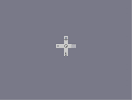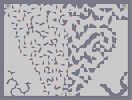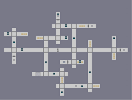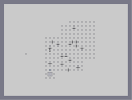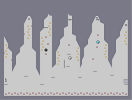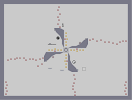Simple Challenge: Laser Snake and Crane Electrocution maze Out of this world. All the things he done Pinwheel

Pages: (2) [ 1 ] 2

### It still pisses me off

that you didn't get a 5! >X(

### HOW THE HELL

DID THIS NOT GET A 5??!!!?!?!
THAT PISSES ME OFF! DX<

the hair.......

### this is insanely good

in full view as well >.< 5aved

### Holy God

the hairs amazing

.

Nice n-art.

### ahhhhhhhh

i love oh so much.

### mate

be careful what you say to people... cause I, for one could do this good...
I still think this art is good however
anyone who seys this is bad, shut up! i bet you could never do something to even COMPARE with this, this is a 6/5 so rate!!!!

### why

do u think ur bad at N its amazing sheesh

### Ha

I swear all the time and I'm 21. Something to get used to if you want to enhance your social life with older people. If you call a bunch of drunken pub blokes (which is what most of my mates are) and tell em they're tossers because they're calling Argentina a bunch of cunts, then you'll get your head kicked in.

Football + Beer = Lots of swearing and shouting.

tossers...

### wow

the hairs amazing how long did u spend on this?
omg, palemoon, you kill me

### Excellent n-art!

I personally think this looks tremendous. Great work!

### excatly unknown

maybe because they were told not to and CHILDREN always do as their told not to do.

### 5

lol.i don't understand why ppl like swearing so much

### you guys are a bunch of tossers

I find swearing rather offensive... I like a lot of anime, but some of it is rather screwed up... swearing does not make you look cooler or older... it is the crutch of human illiteracy... something I am close to overcoming...
now shut the hell up

### I would have

liked to see his hair look more like the one from the comics. Otherwise a great homage to an awesome villain.

### dude

and the n-art is good, no more, no less

### again

and this is the best N art i've ever seen :P

### hey, thank you

thanks for the rating and the tipp
a question, how can i watch your replay of my vid?

nice day

5

look?

<--- look.

### well its been

14 hours,
32 rates,

i enjoyed my time on the top a lot, thanks all again =D.

### lol

im working on my next map (another n-art), and that hair looks excatly like spaghetti XD.

but dont worry, itll be (a ton of) days before i post that =P.

and sorry maxson924 =( i didnt mean to sound like i was forcing my opinions on people (i cant/dont want to).

!!!

### Oh, btw

I'm Christian, and I still curse. Religion honestly doesn't mean as much as some people think when it comes to this stuff.

### If you don't want to curse, fine.

But don't tell others how to talk; it's just plainly aggravating :/

### im agnostic

and i still dont curse.

### But swearing does not make you look any more mature...

I'm a Christian so I guess that may be why I don't tend to swear a lot.

### But erm honestly.

Don't. Unless you want your arse kicked. I'm being serious.

### Sounds like my Sundays.

Honestly. Getting drunk on Sundays is such a bad idea, yet there I am, wanting to go, play guitar, then I come back rat-arsed and angry. Haha.